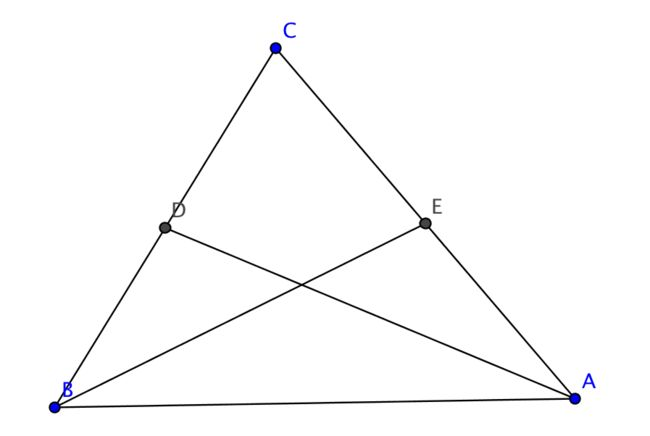# Trigonometry! #10

Geometry Level 2Points $D$ and $E$ are the midpoints of sides $BC$ and $CA,$ respectively, of $\triangle ABC.$

If $AD=5$ and $BC=BE=4,$ then find the length of $CA.$

×

Problem Loading...

Note Loading...

Set Loading...